# 4th Grade Math Worksheets: Fractions

## Fractions worksheets

Our grade 4 fractions worksheets cover addition and subtraction of fractions and mixed numbers, comparing fractions (proper and improper), equivalent fractions and converting mixed numbers to and from improper fractions.

Add like fractions 1/3 + 2/3 =

Add fractions and mixed numbers 1/3 + 2 2/3 =
Add mixed numbers 1 1/3 + 2 2/3 =

Completing whole numbers (improper fractions) 7/2 + ___ = 8
Completing whole numbers (mixed numbers) 3  1/2 + ___ = 8

## Fractions subtraction (like denominators)

Subtract like fractions 2/3 - 1/3 =

Subtract fractions from whole numbers 20 - 4/5 =
Subtract fractions from mixed numbers 2 3/4 - 1/4 =

Subtract mixed numbers from whole numbers 4 - ___ = 1 7/11
Subtract mixed numbers 4 2/3 - 1 1/3 =
Subtract mixed numbers (missing number) 4 2/3 - ___ = 3 1/3

## Convert fractions to/from mixed numbers

Mixed numbers to fractions 3 2/3 =
Mixed numbers to fractions (harder) 17 6/17 =

Fractions to mixed numbers 11/3 =
Fractions to mixed numbers (harder) 37/12 =

## Comparing fractions

Compare two fractions with pie charts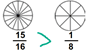Compare two improper or proper fractions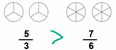Compare mixed numbers and fractions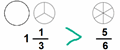Compare two fractions 1/2  >  1/4
Compare two proper or improper fractions 8/7  >  1/2

Ordering 3 fractions 6/7 < 6/5 < 6/2

## Equivalent fractions

Color equivalent fractions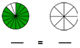Write equivalent fractions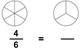Equivalent fractions, (missing numbers) 3/4 = ___ /12
Equivalent fractions, (proper and improper fractions) 5/4 = ___ /20

## Fractional part of a set

Fractional part of a set 1 /3 of 9 = ___

## Fraction word problems

Write and compare fractions word problems Word problems
Add and subtract fractions word problems Word problems
Multiply fractions by whole numbers Word problems